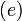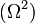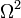# User:Tohline/AxisymmetricConfigurations/Storyline

(Difference between revisions)
 Revision as of 18:28, 5 July 2019 (view source)Tohline (Talk | contribs) (→Storyline)← Older edit Revision as of 18:43, 5 July 2019 (view source)Tohline (Talk | contribs) (→Storyline)Newer edit → Line 21: Line 21: Once you have learned how to construct spherically symmetric, equilibrium self-gravitating configurations from gases that obey a variety of different equations of state, it is natural to ask how those structures will be modified if they are rotating.  You might naturally ask, as well, how techniques that you have learned to use to examine the stability of each spherically symmetric, equilibrium configuration — principally, linear stability analyses and free-energy analyses — might be extended to permit you to examine the stability of rotating equilibrium structures. Once you have learned how to construct spherically symmetric, equilibrium self-gravitating configurations from gases that obey a variety of different equations of state, it is natural to ask how those structures will be modified if they are rotating.  You might naturally ask, as well, how techniques that you have learned to use to examine the stability of each spherically symmetric, equilibrium configuration — principally, linear stability analyses and free-energy analyses — might be extended to permit you to examine the stability of rotating equilibrium structures. - For more than 250 years the astrophysics (and mathematics) community has understood what set of equations must be solved simultaneously in order to build equilibrium models of rotating, self-gravitating gases.  The first — and still extraordinarily relevant — publication was the book by [[User:Tohline/Apps/MaclaurinSpheroids/GoogleBooks#Excerpts_from_A_Treatise_of_Fluxions|Colin Maclaurin (1742) titled, ''A Treatise of Fluxions'']]. + For more than 250 years the astrophysics (and mathematics) community has understood what set of equations must be solved simultaneously in order to build equilibrium models of rotating, self-gravitating gases.  The first — and still extraordinarily relevant — publication was the book by [[User:Tohline/Apps/MaclaurinSpheroids/GoogleBooks#Excerpts_from_A_Treatise_of_Fluxions|Colin Maclaurin (1742) titled, ''A Treatise of Fluxions'']] in which he enumerated the equilibrium properties of a sequence of uniformly rotating, incompressible (polytropic index, n = 0) models . + + +
+ + "The Maclaurin sequence is a sequence of oblate spheroids along which the eccentricity [itex]~(e)[/itex] of the meridional sections increases from zero to one.  A feature of the sequence which caused considerable surprise, at the time it was discovered by D'Alembert, is that along the sequence the square [itex]~(\Omega^2)[/itex] of the angular velocity of rotation is not a parameter of unrestricted range; and that for each value of [itex]~\Omega^2[/itex], less than a certain determinate maximum, there are two permissible spheroidal figures of equilibrium.  It was indeed this last circumstance that led Jacobi first to suspect, and then to verify, that a sequence of genuine tri-axial ellipsoids of equilibrium branches off from the Maclaurin sequence." +
+ — Drawn from §2a of [https://ui.adsabs.harvard.edu/abs/1964ApNr....9..323C/abstract S. Chandrasekhar & N. R. Lebovitz (1964)], Astrophysica Norvegica, 9, 232 +

# (Initially) Axisymmetric Configurations

 "As a practical matter, discussions of the effect of rotation on self-gravitating fluid masses divide into two categories: the structure of steady-state configurations, and the oscillations and the stability of these configurations." — Drawn from N. R. Lebovitz (1967), ARAA, 5, 465 We add a third category, namely, the nonlinear dynamical evolution of systems that are revealed via stability analyses to be unstable.

## Storyline

Once you have learned how to construct spherically symmetric, equilibrium self-gravitating configurations from gases that obey a variety of different equations of state, it is natural to ask how those structures will be modified if they are rotating. You might naturally ask, as well, how techniques that you have learned to use to examine the stability of each spherically symmetric, equilibrium configuration — principally, linear stability analyses and free-energy analyses — might be extended to permit you to examine the stability of rotating equilibrium structures.

For more than 250 years the astrophysics (and mathematics) community has understood what set of equations must be solved simultaneously in order to build equilibrium models of rotating, self-gravitating gases. The first — and still extraordinarily relevant — publication was the book by Colin Maclaurin (1742) titled, A Treatise of Fluxions in which he enumerated the equilibrium properties of a sequence of uniformly rotating, incompressible (polytropic index, n = 0) models .

 "The Maclaurin sequence is a sequence of oblate spheroids along which the eccentricity$~(e)$ of the meridional sections increases from zero to one. A feature of the sequence which caused considerable surprise, at the time it was discovered by D'Alembert, is that along the sequence the square$~(\Omega^2)$ of the angular velocity of rotation is not a parameter of unrestricted range; and that for each value of$~\Omega^2$, less than a certain determinate maximum, there are two permissible spheroidal figures of equilibrium. It was indeed this last circumstance that led Jacobi first to suspect, and then to verify, that a sequence of genuine tri-axial ellipsoids of equilibrium branches off from the Maclaurin sequence." — Drawn from §2a of S. Chandrasekhar & N. R. Lebovitz (1964), Astrophysica Norvegica, 9, 232